# Form of… An Innacurate Prediction!

Activating Transformative Powers in Neural Networks

Before we move into the activation function part of the show, let’s stop and consider what we’ve done in the first step. Applying weights and a bias value to variables/features in a data set is, in effect, making a prediction. We know this prediction is wrong because it’s based on random weight and bias values, but we’ve seen this movie before, a couple of times actually. When we looked at Gradient Boosted Trees, we started with a set of known bad predictions built from a shallow decision tree. With Logistic Regression, we also started with known bad weights and bias values (all 0’s). In both cases, we used a form of gradient descent to slowly adjust themselves until the predictions they generated were as close to the training data as reasonable with the hopes of avoiding overfitting. Thus, if you’ve been paying attention and understand what’s happening at each step in the neural network process, you can probably predict what’s going to happen at some point. :)

That said, we’re not quite there yet. The power of neural networks — like gradient boosted trees — is in their ability to fit more complex shapes. When using y = wx+b for predictions, you end up with a straight line. We need to do something to these predictions that have been generated to give them the ability to form some interesting shapes. And that’s the purpose of the activation function.

Recall that when we went through our first layer of making the predictions, we ended up with a matrix where each row represented the output of a neuron, with one prediction per data point.

Now it’s time to pass these values into our activation function.

# No Negativity Allowed

The basic ReLU activation node is going to perform a simple transformation. It keeps any prediction greater than 0, and any negative values get raised to 0. You can logically represent this as max(value,0), and you might code this activation function something like this:

# No Twins Allowed

If we were to stop here, we’d have a problem — if you map out all of these values on an xy coordinate plane, you’d have two hinge functions with the same basic shape. Both would have a slope of 0 until you hit the origin (0,0) at which point they would both have a slope of 1.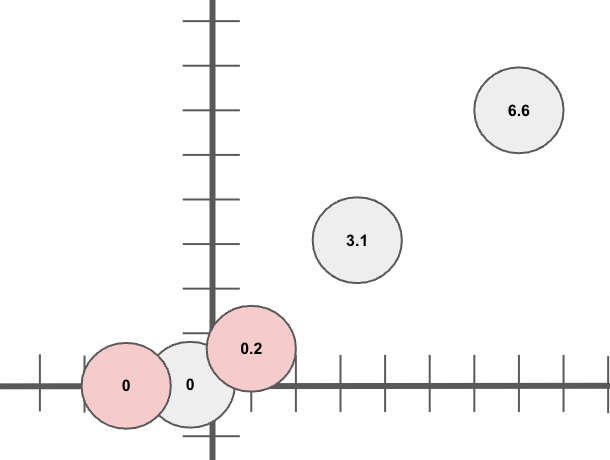Form of… THE SAME FUNCTION! (**yawn**)

Remember, though, that this isn’t the last step in our process. We end up taking this newly transformed dataset and once again put it through a series of weights and bias calculations — in other words, making new predictions from our now transformed predictions.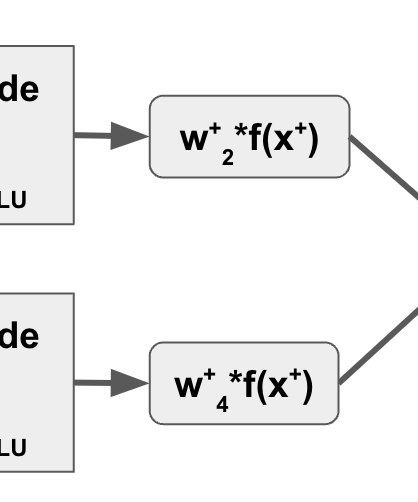**This** is where things get interesting…

# Form Of…

In this scenario, from the first hidden layer on we want to shift our entire function and all values in the same way, so the weight vectors we generate for each neuron are going to be the same value. Thus, instead of using inner products, we can just do a straight multiplication, which allows us to just generate a single weight per neuron.

Since some of those weight values are going to be negative, they’re going to take the positive slope and switch it negative. This weight value is going to change the slope of the line as well. Let’s take a look at this intermediate step:

Now that the slopes for each neuron have been adjusted, we create a random offset to each prediction as well and add it in.

The functional result (pun intended) of this is to give a different output (prediction) based on the prediction before — or in other words, our y values from the previous step now become our x coordinates, and the transformed values are the new y values. This results in a couple of functions that have different, more interesting shapes: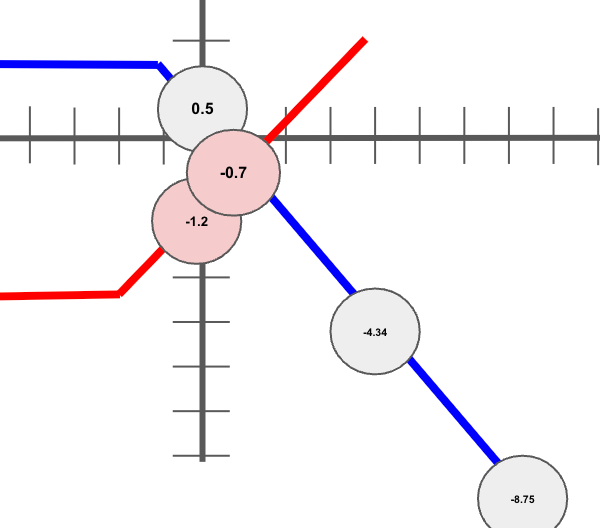Not drawn to scale. If you’re surprised by this, you must be new around here.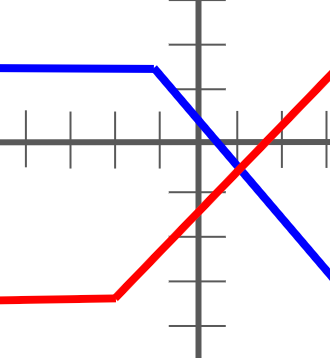Now we’re on to something!

# In Summary

What we’ve done so far in each neuron:

• Took a set of input data and converted each observation into a prediction
• Transformed that data using ReLU activation
• Further modified the results by applying new weight and bias values

Finally, we generate a prediction for each observation by simply summing our two functions together. Mathematically, that looks like this:

So we now have math that gets us from our original data:

…to a numeric prediction function that visualizes like this:

There’s only one thing I know about these predictions so far: they’re wrong, and probably very, very wrong. We know we’re going to need to adjust our weight and bias values in order to get them closer to the actual training labels. But again, we’ve seen this before! We can use gradient descent to move backwards through our neural network (a step called back propogation) and adjust the weight and bias values such that they line up better with our known training labels — or at least as good as we can get given our relatively simple function shape.

For now, though, we’re going to sit and enjoy for a moment the fact that we’ve walked through a series of calculations in a neural network and produced a prediction based on input data and a whole bunch of incorrect assumptions. Let’s take a breather, and then next time go just a little bit… deeper.

--

--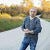## Jason Eden

Data Science & Cloud nerd with a passion for making complex topics easier to understand. All writings and associated errors are my own doing, not work-related.